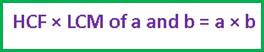## Highest Common Factor HCF

What you will learn in this lesson on HCF

Highest Common Factor (HCF)

What length of a staff will measure two planks of length 36 cms and 48 cms exactly?

It is a 12 cms long staff.

How to find?

In one way, like this:

Factors of 36 are 1, 2, 3, 4, 6, 9, 12, 18, 36, and

Factors of 48 are 1, 2, 3, 4, 6, 8, 12, 24, and 48.

Among the above factors of the two numbers, the highest factor common to both is 12.

In another easier way, it is

36 = 12 × 3, and 48 = 12 × 4.

12 is the greatest factor common to 36 and 48.

Therefore, the two planks 36 and 48 cms long are divided into 3 and 4 pieces respectively, each of length 12 cms.

So, 12 divides both 36 and 48 exactly.

We say, 12 is the highest common factor of 36 and 48.

Now,

36 = 4 × 9 = 22 × 32 and 48 = 3 × 16 = 24 × 3

Now, 2 and 3 are the common prime factors of 36 and 48.

Next, to find the HCF (highest common factor) of 36 and 48, find the product of the least powers of the common prime factors 2 and 3.

It is 22 × 3 = 4 × 3 = 12.

So, there we have, 12 is the highest common factor of 36 and 48

This is called prime factorization of two numbers, i.e. expressing numbers as product of their prime factors.

Prime factorization is a method for finding the HCF (highest common factor) of two numbers.

How to Find the HCF of two or more numbers:

How to Find HCF of Fractions:

HCF  × LCM = a × bFind the HCF of 2/3 and 3/4.

Solution:

HCF of numerators 2 and 3 is 1, and

LCM of denominators 3 and 4 is 12.

Therefore, HCF of 2/3 and 3/4 is 1/12Consider two numbers 12 and 18.

12 = 22 × 3, and 18 = 2 × 32

Therefore, HCF of 12 and 18 is = 2 × 3 = 6, and

LCM of 12 and 18 is = 22 × 32 = 36

Again, product of 12 and 18 is 12 × 18 = 216, and

Product of their HCF and LCM is 6 × 36 = 216